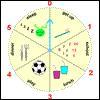#### You may also like### How Many Days?

How many days are there between February 25th 2000 and March 11th?### Planet Plex Time

On Planet Plex, there are only 6 hours in the day. Can you answer these questions about how Arog the Alien spends his day?### On What Day Did it Happen?

Read this article to find out the mathematical method for working out what day of the week each particular date fell on back as far as 1700.

# Stop the Clock for Two

##### Age 5 to 7 Challenge Level:

Here’s a game to play with an adult!How do you play?
You'll need an adult to play with.
You’ll also need a set of blank clock faces, or you could use the interactive version.

Set the time on the clock to $6$ o’clock to start the game.
Starting with the adult, take it in turns to move the hands of the clock on by $\frac{1}{2}$ hour or $1$ hour. For example, the adult could choose $\frac{1}{2}$ hour, so the clock hands move to 6.30, then you might choose $1$ hour, moving the clock hands to 7.30, and so on.
The winner is the person who moves the hands exactly onto $12$ o’clock.

Does it make a difference if you go first instead?
What happens if the adult gets to 10.30? Why?
Can you work out a winning strategy?
Easier version: start at $9$ o’clock instead of $6$ o’clock.
Harder version: try using different time intervals e.g. $\frac{1}{4}$, $\frac{1}{2}$ or $\frac{3}{4}$ hour.